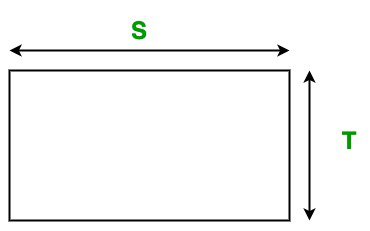Open in App
Not now

• Difficulty Level : Medium
• Last Updated : 08 Sep, 2022

A rectangle is a simple flat figure in a plane. It has four sides and four right-angles. In a rectangle all four sides are not of equal length like a square, sides opposite to each other have equal length and both the diagonals of the rectangle have equal length.

Method overloading allows different methods to have the same name, but different signatures where the signature can differ by the number of input parameters or type of input parameters or both.

#### Area of the Rectangle

The area of the rectangle is the product of its length and width/breadth. We can simply calculate the area of the rectangle using the following formula:Formula:

`Area of the rectangle: A = S * T`

Here, S is the length of the rectangle and T is the breadth/width of the rectangle.

Below is the implementation of the above approach:

## Java

 `// Java program to find the area of``// the rectangle using Method Overloading``import` `java.io.*;` `class` `Rectangle {` `    ``// Overloaded Area() function to``    ``// calculate the area of the rectangle``    ``// It takes two double parameters``    ``void` `Area(``double` `S, ``double` `T)``    ``{``        ``System.out.println(``"Area of the rectangle: "``                           ``+ S * T);``    ``}` `    ``// Overloaded Area() function to``    ``// calculate the area of the rectangle.``    ``// It takes two float parameters``    ``void` `Area(``int` `S, ``int` `T)``    ``{``        ``System.out.println(``"Area of the rectangle: "``                           ``+ S * T);``    ``}``}` `class` `GFG {` `    ``// Driver code``    ``public` `static` `void` `main(String[] args)``    ``{` `        ``// Creating object of Rectangle class``        ``Rectangle obj = ``new` `Rectangle();` `        ``// Calling function``        ``obj.Area(``20``, ``10``);``        ``obj.Area(``10.5``, ``5.5``);``    ``}``}`

Output

```Area of the rectangle: 200
Area of the rectangle: 57.75```

Time Complexity: O(1)

Auxiliary Space: O(1) because constant variables are used

My Personal Notes arrow_drop_up